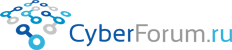Konst2016
 Войти Регистрация Восстановить пароль
 Правила Карта Блоги Социальные группы Поиск Сообщения за день
Оценить эту запись

# Простой автоэнкодер создает картинки с числами

Запись от Konst2016 размещена 12.09.2021 в 20:31
Обновил(-а) Konst2016 15.09.2021 в 15:59 (изм.)
Метки

Здравствуйте! В этой статье я хочу показать как сделал автоэнкодер, что это?Модель обучается на данных mnist
, модель сделана как 784 нейрона входные->128 нейрона->64->49<здесь сеть сужается>->64->784<видно количество входов==количество выходов-это
автоенкодер>, видно модель симметричная, так она может создавать примерно то на чем обучалась, набор X_test создает картинки.
Обучал в Google Colab, сделал сохранение весов и модели свое так у меня theano, а там tensorflow, скачивал pkl файл с левой панели Colab.
Чтобы обучать убрать комментарии, а тот блок закоментировать.
Python
 ```1 2 3 4 5 6 7 8 9 10 11 12 13 14 15 16 17 18 19 20 21 22 23 24 25 26 27 28 29 30 31 32 33 34 35 36 37 38 39 40 41 42 43 44 45 46 47 48 49 50 51 52 53 54 55 56 57 58 59 60 61 62 63 64 65 66 67 68 69 70 71 72 73 74 75 76 77 78 79 80 81 82 83 84 85 86 87 88 89 90 91 92 93 94 95 96 97 98 99 100 101 102 103 104 105 106 107 108 109 110 111 112 113 114 115 116 117 118 119 120 121 122 123 124 125 126 127 128 129 130 131 132 133 134 135 136 137 ``` ```import sys import numpy as np import matplotlib.pyplot as plt import sys import os import keras from keras.layers import Input, Flatten, Reshape, Dense import pickle from PIL import Image import gzip     fpickle='learned.pkl'     def load_dataset():     # We first define a download function, supporting both Python 2 and 3.     if sys.version_info == 2:         from urllib import urlretrieve     else:         from urllib.request import urlretrieve       def download(filename, source='http://yann.lecun.com/exdb/mnist/'):         print("Downloading %s" % filename)         urlretrieve(source + filename, filename)       # We then define functions for loading MNIST images and labels.     # For convenience, they also download the requested files if needed.           def load_mnist_images(filename):         if not os.path.exists(filename):             download(filename)         # Read the inputs in Yann LeCun's binary format.         with gzip.open(filename, 'rb') as f:             data = np.frombuffer(f.read(), np.uint8, offset=16)         # The inputs are vectors now, we reshape them to monochrome 2D images,         # following the shape convention: (examples, channels, rows, columns)         data = data.reshape(-1, 1, 28, 28)         # The inputs come as bytes, we convert them to float32 in range [0,1].         # (Actually to range [0, 255/256], for compatibility to the version         # provided at http://deeplearning.net/data/mnist/mnist.pkl.gz.)         return data / np.float32(256)         def load_mnist_labels(filename):         if not os.path.exists(filename):             download(filename)         # Read the labels in Yann LeCun's binary format.         with gzip.open(filename, 'rb') as f:             data = np.frombuffer(f.read(), np.uint8, offset=8)         # The labels are vectors of integers now, that's exactly what we want.         return data     # We can now download and read the training and test set images and labels.     X_train = load_mnist_images('train-images-idx3-ubyte.gz')     y_train = load_mnist_labels('train-labels-idx1-ubyte.gz')     X_test = load_mnist_images('t10k-images-idx3-ubyte.gz')     y_test = load_mnist_labels('t10k-labels-idx1-ubyte.gz')       # We reserve the last 10000 training examples for validation.     X_train, X_val = X_train[:-10000], X_train[-10000:]     y_train, y_val = y_train[:-10000], y_train[-10000:]       # We just return all the arrays in order, as expected in main().     # (It doesn't matter how we do this as long as we can read them again.)     return X_train, y_train, X_val, y_val, X_test, y_test     def build_model():   input_img=Input((1, 28, 28))     x=Flatten()(input_img)     x=Dense(128, activation='relu')(x)   x=Dense(64, activation='relu')(x)   encoded=Dense(49, activation='relu')(x)     d=Dense(64, activation='relu')(encoded)   d=Dense(28 * 28, activation='sigmoid')(d)   decoded=Reshape((1, 28, 28))(d)     autoencoder=keras.Model(input_img, decoded, name='autoencoder')     return autoencoder     def make_autoencoder(fdump='', create_via_file=False):     model=build_model()     if create_via_file:         params={}         with open(fdump, 'rb') as f:            params=pickle.load(f)         len_layers=len(model.layers)           for i in range(len_layers):             model.layers[i].set_weights(params[i])       return model             def main():   X_train, y_train, X_val, y_val, X_test, y_test = load_dataset()       model=make_autoencoder(fdump=fpickle, create_via_file=True)   n=10   decoded_imgs=model.predict(X_test[:n,], batch_size=n)     for i in range(n):           img_t=decoded_imgs[i].squeeze()       img_t[img_t>=0.5]=255       img=Image.fromarray(np.uint8(img_t))       img.save('nn_created'+str(i)+'.png')             #   model=make_autoencoder() #   model.compile(optimizer='adam', loss='mean_squared_error')   #   batch_size=100     #   model.fit(X_train, X_train, #   validation_data=(X_val, X_val), #   epochs=0, #   batch_size=batch_size, #   shuffle=True) #   len_layers=len(model.layers) #   weights={} #   for i in range(len_layers): #       weights[i]=model.layers[i].get_weights()   #   with open(fpickle, 'wb') as f: #       pickle.dump(weights, f)     main()```
Вывод:Некоторые неизвестные мне классы/методы:
a.squeeze(axis=None)

Remove axes of length one from `a`.

Refer to `numpy.squeeze` for full documentation.

 Powered by vBulletin® Version 3.8.9Copyright ©2000 - 2021, vBulletin Solutions, Inc.